# CBSE Class 11 Physics Chapter 8 Revision Notes

Chapter 8: Gravitation Revision Notes

## Kepler’s laws of Planetary Motion

Three laws of planetary motion were proposed by Johannes Kepler. The following are the details:

(i) Law of Orbits: Each planet travels in an elliptical orbit around the sun, with the sun at one of the elliptical foci.

(ii) Law of Areas: The planet’s speed varies to the point where the radius, or vector, drawn from the sun to the planet sweeps out equal areas in equal time.

(iii) Law of Periods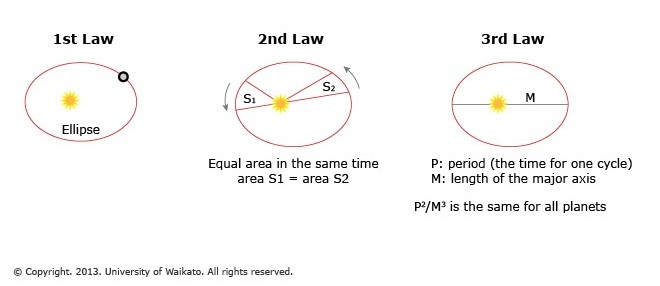Source

## Newton’s Law of Gravitation

• According to Newton’s law of gravitation, every particle in the universe attracts every other particle with a force that is directly proportional to the product of their masses and inversely proportional to the square of their separation. The force is d__irected along the line__ that connects the particles.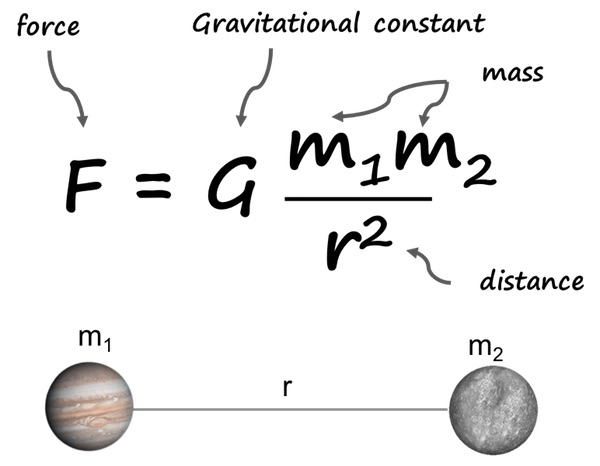Source

• The force of attraction between two particles of equal mass separated by a unit distance is numerically equal to the universal constant of gravitation G.

## Important Gravitational Force Characteristics

(i) The gravitational force between two bodies is a central force, acting along the line connecting the two interacting bodies’ centers.

(ii) The nature of the intervening medium has no bearing on the gravitational force between two bodies.

(iii) The presence of other bodies has no bearing on the gravitational force between two bodies.

(iv) It is valid for spherically symmetrical and point objects.

(v) The magnitude of force is negligible.

### Acceleration Due to Gravity

• Acceleration due to gravity is the acceleration produced in a body as a result of the force of gravity.
• The letter ‘g’ is usually used to represent it. It always points to the center of the Earth.
• The gravitational force acting on a body of mass ‘m’ lying on the earth’s surface is given by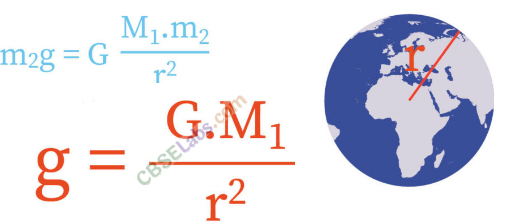Source

### Gravitational Acceleration Variation

• The value of gravity’s acceleration varies with height (i.e., altitude), depth, earth’s shape, and rotation around its own axis.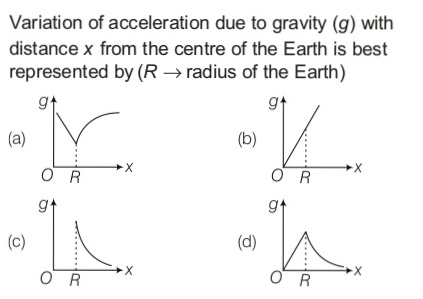Source

### Gravitational Field

• The gravitational field is the space around a body in which its gravitational force of attraction is felt by other bodies.

### Gravitational Field Intensity

• The force experienced by a body of unit mass placed at a point in the gravitational field is defined as the intensity of the gravitational field of that body, assuming that the presence of unit mass does not disturb the original gravitational field.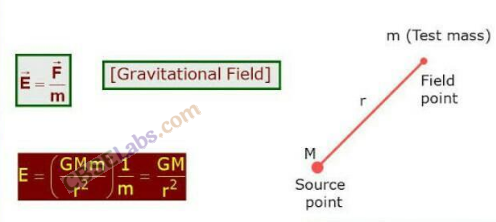Source

## Gravitational Potential

• The amount of work required to bring a body of unit mass from infinity to a point in the gravitational field of a body is defined as the gravitational potential at that point.
• Gravitational Potential Energy is the gravitational potential at a point r from a body or particle of mass M.
• Gravitational potential energy is the work done in transporting a mass ‘m’ from infinity to a point at a distance r.
]]>